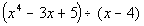We use the synthetic division method when you need to divide a polynomial function by a binomial of the form x - c. We can make the division of the polynomial a little easier and simple by using the synthetic division method. Students can also use the synthetic division calculator to find the solution of the division. By using the synthetic division method we can find the quotient and the remainder of the polynomial. The remainder theorem explains to us that the synthetic division actually gives the functional value of the remainder, which we can use. We can also use synthetic division in finding the factors of a polynomial. The synthetic division is a shortcut method of solving the decision of the polynomials. The synthetic substitution calculator is helpful in solving the division.

In this article, we will try to learn how to use the synthetic division method to solve the division of the polynomial: We will solve the synthetic division is steps:

## Set up for the synthetic division:

The easy way to do the synthetic division is to set the polynomial as you are doing the long division, as it makes you analyze where you have to place the “0” and where you have to bring the coefficient for the division. Now make sure the following steps:

The divisor (the polynomial by which you are dividing) goes outside the box. The dividend( the polynomial you are dividing into) would be written inside the box.

Try to write the dividend in the descending order of the powers, and insert “0” for any missing power.

Now if you have to solve a problem .Now figure out the polynomial is starting out with the degree of “4”. There are missing degrees of 3 and 2. So put both the polynomials in the division box and add “0” in th missing degrees.

You check out the value of the “C”. The divisor polynomial (X-C) having the value of the “C” at the end of the divisor polynomial. Then write the coefficients of the dividend to the right. You also have to add the “0” that was inserted in the missing terms.

Bring down the leading coefficient to the bottom.

Now, multiply the “C” with the leading coefficient, and place the value beneath the next coefficient of the dividend.

Now add the column created in the and write it down as shown in figure

Now repeat these steps until the end of the division.

Now write down the answer. The number in the last row is your coefficient of the quotient as well as the remainder of the division. You can say the final value which is, in this case, is “249” is the remainder of the synthetic division. Now you go from right to left. The next number is your constant, which in this case is “61”, the next would be your value of x, which is “16”. The nest proceeding number would be the coefficient of the square value of x, which is “4”.

It is important to notice the degree of the quotient is one less the degree of the dividend. Now if the degree of the dividend is 4, the degree of the quotient would be 3.

The result of the synthetic division would be:

The synthetic division is a simple method of solving the long division of the polynomial, students can solve the division by using the synthetic division. It is also better to use the synthetic division calculator for solving the division of polynomials. Sometimes, the long division of the polynomial can be long and lengthy and can take a lot of the time of the students to solve a problem. The synthetic division calculator can make the question a little easier for you.

Submit a Guest post is the way to rank your blog on Google Search Engine. Email: business.glimpse.info@gmail.com.